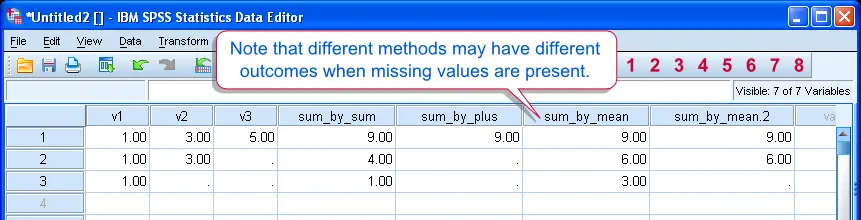SPSS TUTORIALS BASICS ANOVA REGRESSION FACTOR CORRELATION

# Computing Sums in SPSS – 3 Easy Options

In SPSS, `SUM(v1,v2)` is not always equivalent to `v1 + v2`. This tutorial explains the difference and shows how to make the right choice here.Different Ways of Taking Sums have Different Outcomes when Missing Values are Present

## Explanation

• In SPSS, `v1 + v2 + v3` will result in a system missing value if at least one missing value is present in v1, v2 or v3.
• The first alternative, `SUM(v1, v2, v3)` implicitly replaces missing values with zeroes.
• The second alternative, `MEAN(v1, v2, v3) * 3` implicitly replaces missing values with the mean of the non missing values.
• The third alternative, `MEAN.2(v1, v2, v3) * 3` is almost similar to the second. However, by suffixing `MEAN` by `.2`, you ensure that a mean is only calculated if at least two non missing values are present in v1, v2 and v3.
• These points are demonstrated by the syntax below.

## SPSS Syntax Demonstration

data list free/v1 v2 v3.
begin data
1 3 5
1 3 ''
1 '' ''
end data.

compute sum_by_sum = sum(v1,v2,v3).
compute sum_by_plus = v1 + v2 + v3.
compute sum_by_mean = mean(v1 to v3) * 3.
compute sum_by_mean.2 = mean.2(v1 to v3) * 3.
exe.

## So Which one Is Best?

• This question is rather hard to answer. It may depend on the meaning of the missing values (question skipped? technical problem?). Also, what are the individual questions and the sum supposed to reflect?
• Second, the amount of missing values and sample size may be taken into account. Does it permit excluding some observations with missing values? Will this affect representativity and if so, is that a real problem?
• For one thing, sums calculated by `SUM` may be biased towards zero. For instance, if v1 through v3 measure components of satisfaction, respondents will be seen as "less satisfied" insofar they have more missing values. That conclusion may be misleading.
• Using the `+` operator does not induce such bias but may result in many missing values in the sum. This problem becomes larger as more missing values are present in the input variables and a sum is taken over more variables.
• Multiplying the mean by the number of variables, may be a better alternative. However, it will always come up with a sum if there's at least one non missing value. Especially with many input variables, a single value may be jugded insufficient for inferring a summation measure.
• But perhaps none of these options is expected to yield sufficiently accurate results. In this case, one could partly circumvent the problem with a (multiple) imputation of missing values.

# Tell us what you think!

*Required field. Your comment will show up after approval from a moderator.

# THIS TUTORIAL HAS 4 COMMENTS:

•### By Mengesha Abrha on January 6th, 2017

This tutor is very fantastic and still we want to explore more. thanks in advance

•### By Vu on November 14th, 2018

Super page. The way you explained things is so simple but easy to understand. Thank so much!

•### By Clare on January 11th, 2021

Is this still true? It seems like even SUM won't work if there are missing values now.

•### By Ruben Geert van den Berg on January 12th, 2021

Hi Clare,

SPSS is extremely careful about backwards compatibility: syntax that ran in older versions usually runs (exactly similarly) in newer versions. I can't come up with a single exception to this rule.

But anyway, I just tested the syntax below in SPSS 27 and everything still runs the same.

DATA LIST FREE/V1 V2 V3.
BEGIN DATA
1 '' 3
END DATA.

COMPUTE SUMA = SUM(V1 TO V3).
COMPUTE SUMB = V1 + V2 + V3.
COMPUTE SUMC = MEAN(V1 TO V3) * 3.
EXECUTE.

Perhaps you've a different problem such as all values specified as user missings or string variables?

Hope that helps!

SPSS tutorials